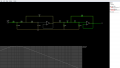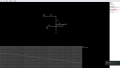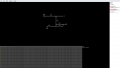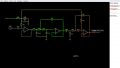# Calculating Coupling and Decoupling Capacitors

#### MrAl

Joined Jun 17, 2014
6,821
Yeah, i know. I have to use a voltage divider to get Half the supply voltage and then apply it to the opamps input. The other input then has to have a coupling capacitor, then it should work just fine. Another coupling capacitor has to be used At the output of the opamp to remove the dc Offset. I think, the higher the value for the decoupling capacitor, the more clean the dc supply voltage will get. But what value should be enough to decouple a 24v / 12v SMpsu voltage?
Hi,

The old standard for digital logic was a 10uf in parallel with a 0.1uf.
However, it really depends on the current changes and the impedance of the power supply being used. You could need as high as 1000uf (low ESR) in parallel with 0.1uf.

#### Audioguru again

Joined Oct 21, 2019
546
A switching power supply uses a high frequency that is difficult to remove. A high value decoupling capacitor filters away low frequencies well but has inductsnce that causes poor filtering at high frequencies. Also, opamps have poor power supply noise rejection at high frequencies.

#### Yeye

Joined Nov 12, 2019
47
But a Capacitor in parallel means, it acts as a low pass filter. The resistence is very low actually. The noise is high Frequency noise, because of the switching process in that power supply. High capacitor value means low cutoff frequency ( Should actually be quite low, because the series resistence should be high, since the opamps power supply line draws low current. The divided supply voltage is being used at the input of the opamp, which has really high impedance -> The decoupling Capacitor there doesnt have to be highly capacitive.)
Another Factor, that might change some things, is the following:
The power supply is being used for the main amplifier board and for the Filter circuit in parallel. So the capacitor value does have to be high? It will supply around 5A rms, since the output at the Speaker is around 100W rms and the PSU is a 24v one. Then the resistence would equal around 4 ohms ( 4,8 ohms RMS ), with a 1000uF capacitor, i would achieve a cutoff Frequency 40hz (-3db).
Is that enough? The capacitors inductance then is quite high for a capacitor, but that should not matter right? I mean it only acts like a high pass Filter, but since the frequencies there already are heaviely weakened, because of the Capacitor itself, this should work, shouldn't it?

#### MrAl

Joined Jun 17, 2014
6,821
The modern way to 'filter' the output of a switcher is to use a small value inductor in series and a large value capacitor across the very output. So the inductor is in series with the cap and the output is now taken from across the cap.
The feedback however is left alone and NOT moved to the very output but left on the node that was previously the output.

It's amazing how much noise this LC filter can remove even with a small hand wound air core inductor like 2uH.
With 2uH and 1000uf the switch frequency of 50kHz (typical) is taken down to 1/2000 of its original amplitude.

#### Audioguru again

Joined Oct 21, 2019
546
Have you tired the switching power supply with the class-D (switching) power amplifier to hear if there is interference from the high switching frequencies beating together?

#### Yeye

Joined Nov 12, 2019
47
Yes, of course i tried. There is no noise, or at least, I can't hear any noise at the output of the Amplifier Board.

#### Audioguru again

Joined Oct 21, 2019
546
Yes, of course i tried. There is no noise, or at least, I can't hear any noise at the output of the Amplifier Board.
Then either you are lucky or you are old like me. My hearing aids fix my normal-for-my-age high frequency hearing loss.

#### Yeye

Joined Nov 12, 2019
47
I actually am 15 :_DAt the right Opamp stage, there is no need of an input coupling capacitor, and also no need of an output coupling capacitor of the left stage, right?
I only have to calculate an input capacitor for the left opamp input ( The capacitor will be placed just between the signal and the 9.1k Ohm resistor, this is, how it should work, right? ) and an output coupling capacitor for the right opamp output.

The one at the output of the right stage should be easy to calculate, since an Opamp acts like a buffer. I only dont know whether the Feedback Resistor ( 33k Ohm ) does affect the Calculation of that capacitor. If not, i only have to know the Impedance of the following circuit, but if it does, is the 33k Ohm in Series or in parallel? ( I think it's parallel )

The one at the input will be hard tho, could anyone help me there?

Thank you as always.

EDIT: Where would i have to apply half the supply Voltage? at the inverting input of the opamps or at the non inverting one ( If there, behind/ in front of which component?)?

#### Audioguru again

Joined Oct 21, 2019
546
The output of an opamp circuit is a very low impedance so the 33k feedback resistor has no effect. Calculate the output capacitor for the lower frequency you want into its load resistance. The 160k resistor must connect to half the supply voltage at a low impedance at all frequencies. Use an opamp.

#### Yeye

Joined Nov 12, 2019
47
Okay, i expect the half voltage to be supplied directly at the non inverting input ( the node 43k Ohm and 10nF at the first opAmp and the 160k OHm and 68nF at the Second opAmp ), The output capacitor is at the output of the Second OpAmp, where is the input capacitor tho? ( I think it is just between the non inverting input and the 43k Ohm Resistor, is this right? )
The circuit would then look like this:The +12v Represent half the Supply voltage.
The capacitors with the "UNKNOWN" Text above, represent the coupling capacitors.
Is this circuit correct?
How to calculate the input capacitor? ( Which capacity already is given by that circuit, which resistence is already given? )

#### MrAl

Joined Jun 17, 2014
6,821
Okay, i expect the half voltage to be supplied directly at the non inverting input ( the node 43k Ohm and 10nF at the first opAmp and the 160k OHm and 68nF at the Second opAmp ), The output capacitor is at the output of the Second OpAmp, where is the input capacitor tho? ( I think it is just between the non inverting input and the 43k Ohm Resistor, is this right? )
The circuit would then look like this:
View attachment 193735

The +12v Represent half the Supply voltage.
The capacitors with the "UNKNOWN" Text above, represent the coupling capacitors.
Is this circuit correct?
How to calculate the input capacitor? ( Which capacity already is given by that circuit, which resistence is already given? )

Why do you have the non inverting input connected to +12v directly?
I dont think you can use that circuit as is on a single supply. Consider a slowly changing input sine. The input would have to be biased as well as the op amps. That's not always possible.

Last edited:

#### Audioguru again

Joined Oct 21, 2019
546
Your Falstad schematic is a nightmare of a negative image with dots all over the wires.
I fixed the circuit so that it works from a single +24V supply:

#### Attachments

•DickCappels

#### Yeye

Joined Nov 12, 2019
47
I answered at my other thread, I'm sorry for writing such a long text, but i just want to understand the whole topic, to later not have to ask you again :_D
Thank you for your support Audioguru again
And thanks to the other replieers.

#### Audioguru again

Joined Oct 21, 2019
546
Your graph has no amplitude and frequency numbers.
Your 1k resistor is supposed to be 100k ohms.

#### Audioguru again

Joined Oct 21, 2019
546
Actually, my 22uF filter capacitor is not feeding one 100k resistor, instead it is feeding two 100k resistors so it will be near a full charge in 1.1 seconds.

#### Yeye

Joined Nov 12, 2019
47
Okay, now things look way betterThank you for that correction, i now simulated the "noise" that's going through the half VCC line, and realized it should be like nearly nothing.As you see it is -46db at 10hz... This should be good enough to not really influence the final output signal.This is the filter which is created by the first coupling capacitor. And this one also looks quite Good, which means i would finally be done with this ^^

Thank you so much for helping me!

This is how the final circuit looks like:THe output capacitor will be calculated with the help of the input impedance of the following real amplifier board.

Thank you again

#### Yeye

Joined Nov 12, 2019
47
Okay, 2 LAST questions for sure:

I wondered, why i calculate coupling (series) capacitors with the input Impedance ( Series ), because a Filter consists of a Series and parallel component, so i tried explaining it:
The opamp's inputs are just like probes of a multimeter probing across a resistor ( The resistence of the Wire going from the capacitor to the opamp input )

Can i say in general, The input resistence of an Opamp always adds as parallel resistence?

How does a Feedback component add to the Circuit? Parallel or Series?

Ty

#### DickCappels

Joined Aug 21, 2008
6,012
The answer to that question can only be determined from studying the circuit. Can you post a schematic diagram of your circuit?

#### Yeye

Joined Nov 12, 2019
47
"This is how the final circuit looks like: "

It actually is the circuit I posted here.
The feedback components i meant are for example the Feedback Capacitor ( 68nF ) and the Feedback resistor (33kOhm).

Something is in parallel to something else, if the first lead of the component is connected to the same node as the first lead of the second component and the 2nd lead to the same node as the 2nd lead of the other component.

Since the feedback 68nF capacitor For example only is connected with 1 Lead to the same node as the 10nF capacitor, and the second lead is connected to the Opamp Output, while the second lead of the 10nf capacitor is connected to ground. Are the 68nF capacitor and the 10nF capacitor connected in parallel or in series to each other?

And with my first question, i wanted to ask, how the input Impedance of the Opamp adds to the 43k Ohm resistor or in general to the input rail.
Maybe you will understand what i mean with this question:
If the input impedance of the opamp is 100k Ohm and i have a 50k Ohm resistor in series to the Signal source, is the Impedance (and with that also the Load Resistence) then 150kOhm or is it 33,33kOhm?

#### Janis59

Joined Aug 21, 2017
906
RE: ""Calculating Coupling and Decoupling Capacitors""
There are wonderful new tool for this aim, outside.
Just a week they shown me.
At k-sim.kemet.com
PS, sorry, they have been changing the placing, now k-sim stands at https://ec.kemet.com/design-tools/
Just try it at a glance

Last edited: